JMSLTM Numerical Library 7.2.0
com.imsl.stat

## Class KaplanMeierECDF

• All Implemented Interfaces:
Serializable, Cloneable

```public class KaplanMeierECDF
extends Object
implements Serializable, Cloneable```
Computes the Kaplan-Meier reliability function estimates or the CDF based on failure data that may be multi-censored.

The Kaplan-Meier (K-M) Product Limit procedure provides simple estimates of the reliability function or the CDF based on failure data that may be multi-censored. No underlying probability model is assumed; K-M estimation is an empirical (non-parametric) procedure. Exact times of failure are required.

Consider a situation in which we are reliability testing n (non-repairable) units taken randomly from a population. We are investigating the population to determine if its failure rate is acceptable. In the typical test scenario, we have a fixed time T to run the units to see if they survive or fail. The data obtained are called Censored Type I data.

During the T hours of test we observe r failures (where r can be any number from 0 to n). The failure times are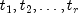, and there are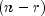units that survived the entire T-hour test without failing. Note that T is fixed in advance, and r is an output of the testing, since we don't know how many failures will occur until the test is run. Note that we assume the exact times of failure are recorded when they occur.

This type of data is also called "right censored" data since the times of failure to the right (i.e., larger than T) are missing. The steps for calculating K-M estimates are the following:

1. Order the actual failure times from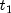through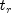, where there are r failures
2. Corresponding to each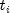, associate the number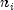with= the number of operating units just before the ith failure occurred at time3. First estimate the survival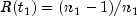4. Estimate each ensuing survival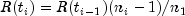,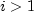5. Estimate the CDF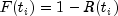,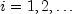Note that non-failed units taken off testing (i.e., right-censored) only count up to the last actual failure time before they were removed. They are included in thecounts up to and including that failure time, but not after.

Example, Serialized Form
• ### Constructor Summary

Constructors
Constructor and Description
`KaplanMeierECDF(double[] t)`
Constructor for `KaplanMeierECDF`.
• ### Method Summary

Methods
Modifier and Type Method and Description
`double[]` `evaluateCDF()`
Computes the empirical CDF and returns the CDF values up to, but not including the time values returned by `getTimes`.
`int` `getNumberOfPoints()`
Retrieves the number of points in the empirical CDF
`double[]` `getTimes()`
Retrieves the time values where the step function CDF jumps to a greater value.
`void` `setCensor(int[] censor)`
Set flags to note right-censoring
`void` `setFrequency(int[] freq)`
Sets the frequency for each entry in `t`
• ### Methods inherited from class java.lang.Object

`clone, equals, finalize, getClass, hashCode, notify, notifyAll, toString, wait, wait, wait`
• ### Constructor Detail

• #### KaplanMeierECDF

`public KaplanMeierECDF(double[] t)`
Constructor for `KaplanMeierECDF`.
Parameters:
`t` - a `double` array containing the failure times.
• ### Method Detail

• #### evaluateCDF

`public double[] evaluateCDF()`
Computes the empirical CDF and returns the CDF values up to, but not including the time values returned by `getTimes`.
Returns:
a `double` array of CDF values.
• #### getNumberOfPoints

`public int getNumberOfPoints()`
Retrieves the number of points in the empirical CDF
Returns:
an `int` containing the number of points in the empirical CDF.
Throws:
`IllegalStateException` - is thrown if the CDF has not been evaluated.
• #### getTimes

`public double[] getTimes()`
Retrieves the time values where the step function CDF jumps to a greater value. This array has right-censored values of `t` removed.
Returns:
a `double` array of time values.
Throws:
`IllegalStateException` - is thrown if the CDF has not been evaluated.
• #### setCensor

`public void setCensor(int[] censor)`
Set flags to note right-censoring
Parameters:
`censor` - an `int` array of 0 or 1 flags to note right-censoring. Values of 0 = continue to use datum and 1 = remove datum. If this method is not called, no data is right-censored.
• #### setFrequency

`public void setFrequency(int[] freq)`
Sets the frequency for each entry in `t`
Parameters:
`freq` - a `double` array containing the repeat count for each entry in `t`.
JMSLTM Numerical Library 7.2.0